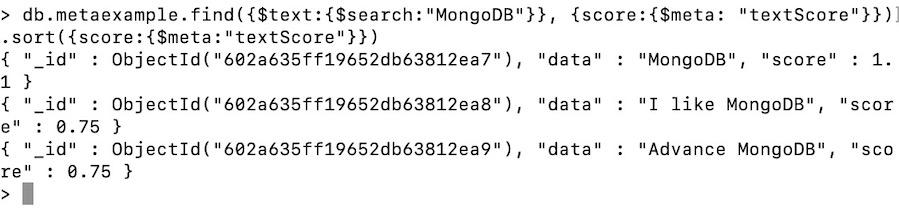Open in App
Not now

# Sorting Documents in MongoDB

• Last Updated : 17 Feb, 2021

Sorting is the way to arrange documents in ascending or descending order. In MongoDB, we can sort documents in ascending or descending order according to field data. To sort documents in a collection we use the sort() method. This method takes a parameter that contains a field: value pair that defines the sort order of the result set, if the value of this field is 1 then this method sorts the documents in ascending order, or if the value of this field is -1 then this method sorts the documents in descending order.

Syntax:

db.Collection_name.sort({field_name : 1 or -1})

Parameter:

This method takes a document that contains a field : value pair. If the value of this field is 1 then this method sorts the documents in ascending order, or if the value of this field is -1 then this method sorts the documents in descending order.

Return:

This method return sorted documents.

Examples:

In the following examples, we are working with:

Database: gfg

Collections: student

Document: Four documents contains name and age of the students.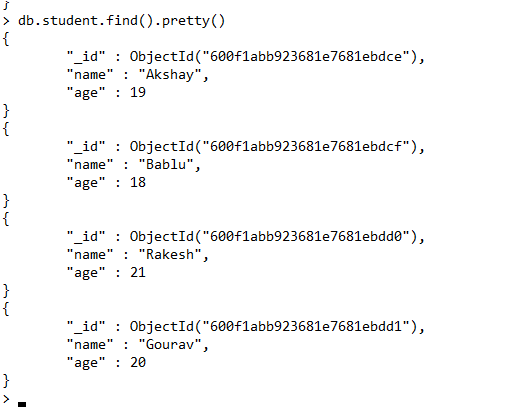• Return all the documents in ascending order of the age:
`db.student.find().sort({age:1})`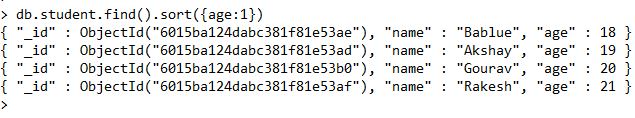• Return all the documents in descending order of the age:
`db.student.find().sort({age:-1})`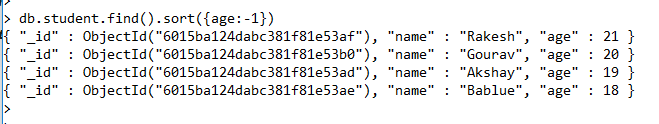## Sorting embedded documents

In MongoDB, we can also sort embedded documents using the sort() method. In this method, we can specify the embedded document field using dot.

Syntax:

db.Collection_name.sort({“field_name.embed_field_name” : 1 or -1})

Example:

In the following example, we are working with:

Database: gfg

Collections: student

Document: Two documents contains name and a marks document, that contains the two subjects marks and the total marks of two subjects.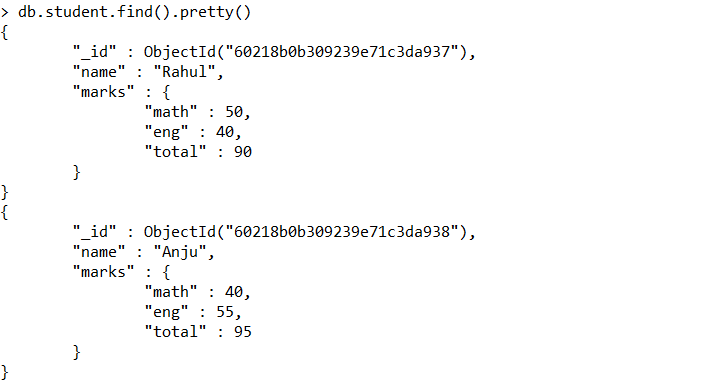• Sort documents in ascending order according to the total field of the marks document:
`db.student.find().pretty().sort({"marks.total":1})`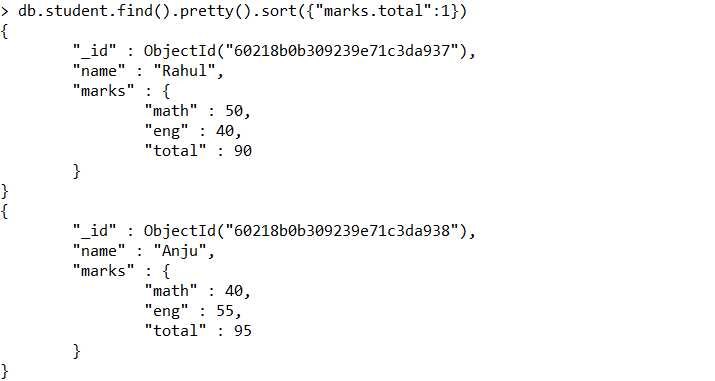• Sort documents in descending order according to the total field of the marks document:
`db.student.find().pretty().sort({"marks.total":-1})`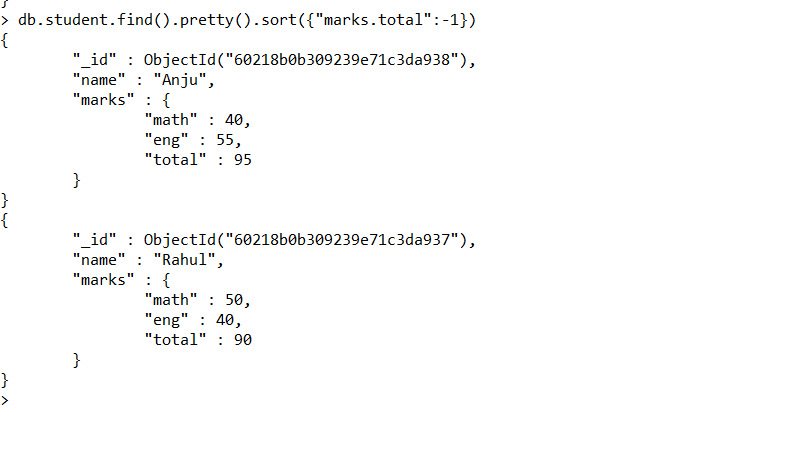## Sorting multiple fields in the documents

In MongoDB, we can also sort multiple fields using sort() method. In this method, you should declare multiple fields that you want to sort. Now this method, sort the fields according to their declaration position, here the sort order is evaluated from left to right.

Syntax:

db.Collection_name.sort({field_name1 : 1 or -1, field_name2 : 1 or -1})

Example:

In the following examples, we are working with:

Database: gfg

Collections: Teacher

Document: Six documents contains the details of the teachers.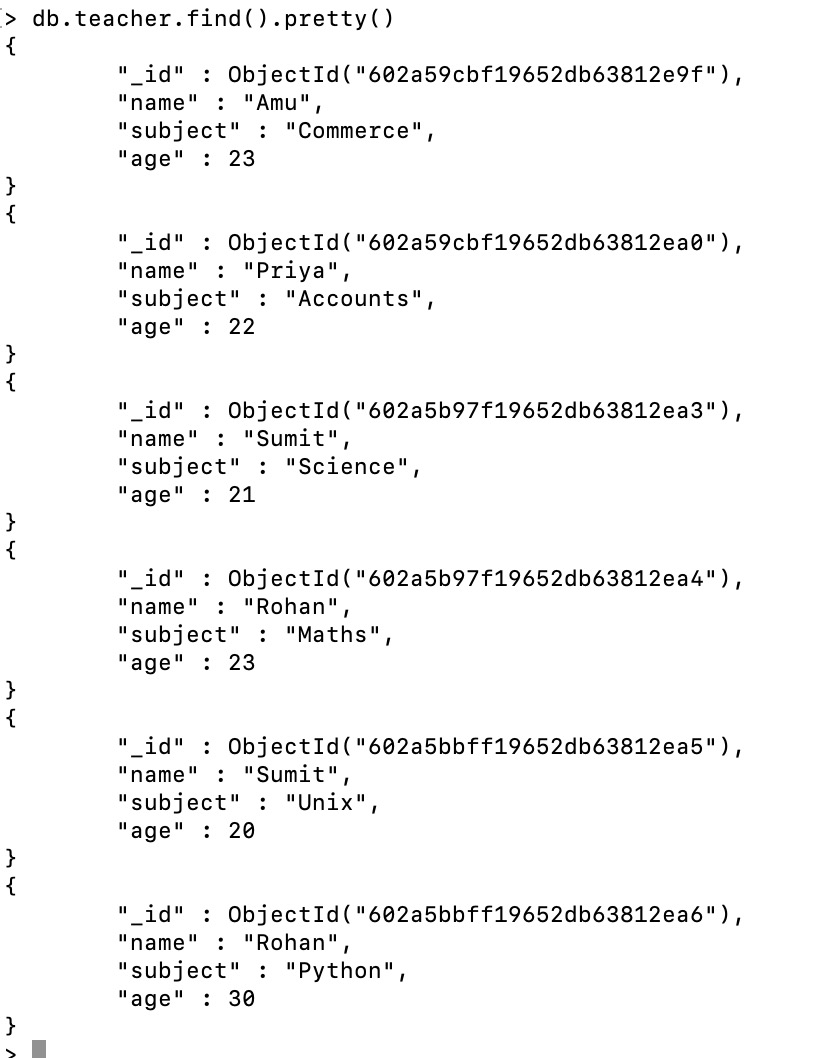Now we sort the documents of the teacher collection in ascending order. Here, we sort multiple fields together using the sort() method(i.e., subject, age)

`db.teacher.find().pretty().sort({subject:1, age:1})`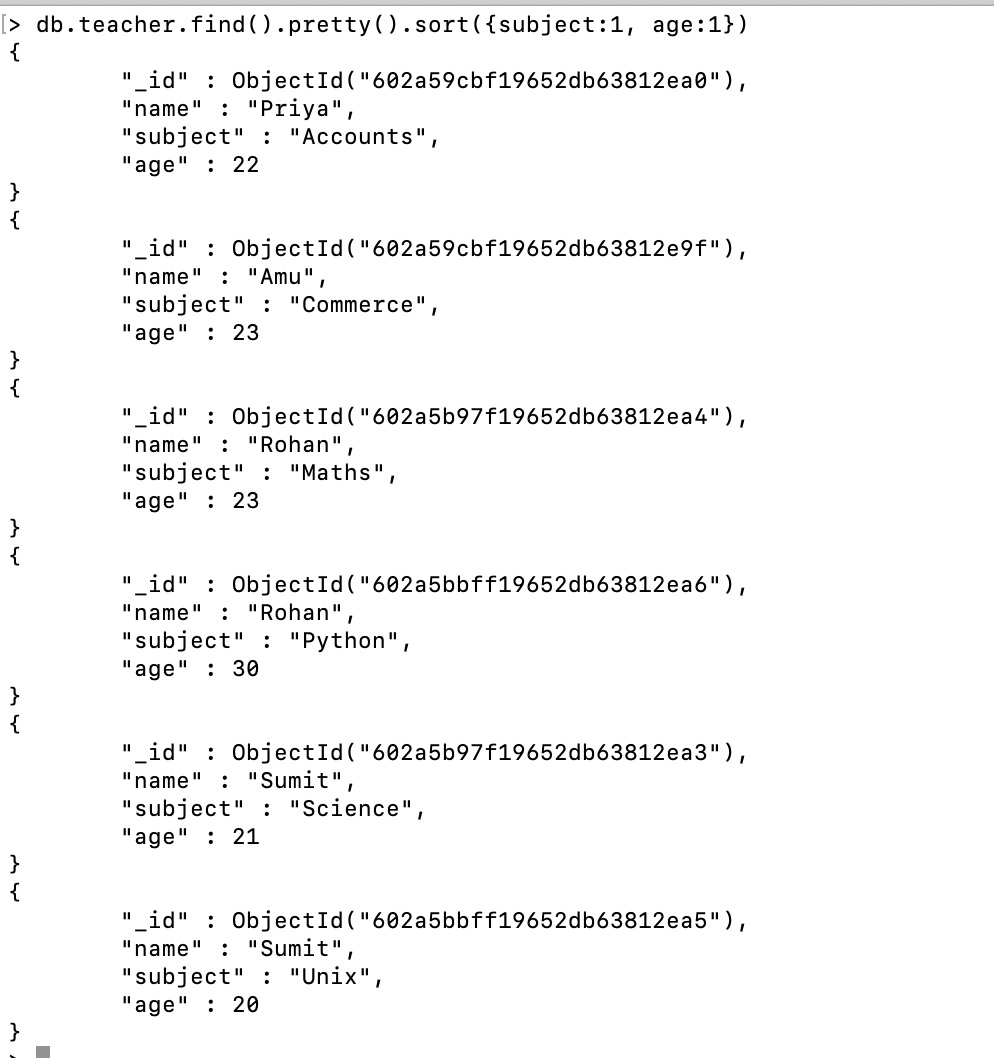## Sorting using the limit() method

In MongoDB, we can limit the number of sorted documents displays in the result using the limit() method. In this method, we specify the number of documents that we want in our result.

Syntax:

db.Collection_name.find().sort({field_name : 1 or -1}).limit(<number>)

Example:

In the following examples, we are working with:

Database: gfg

Collections: teacher

Document: Six documents contains the details of the teachers.Now we sort the documents of the teacher collection in ascending order using the sort() method but in the result, we will only display 4 documents.

`db.teacher.find().pretty().sort({name:1}).limit(4)`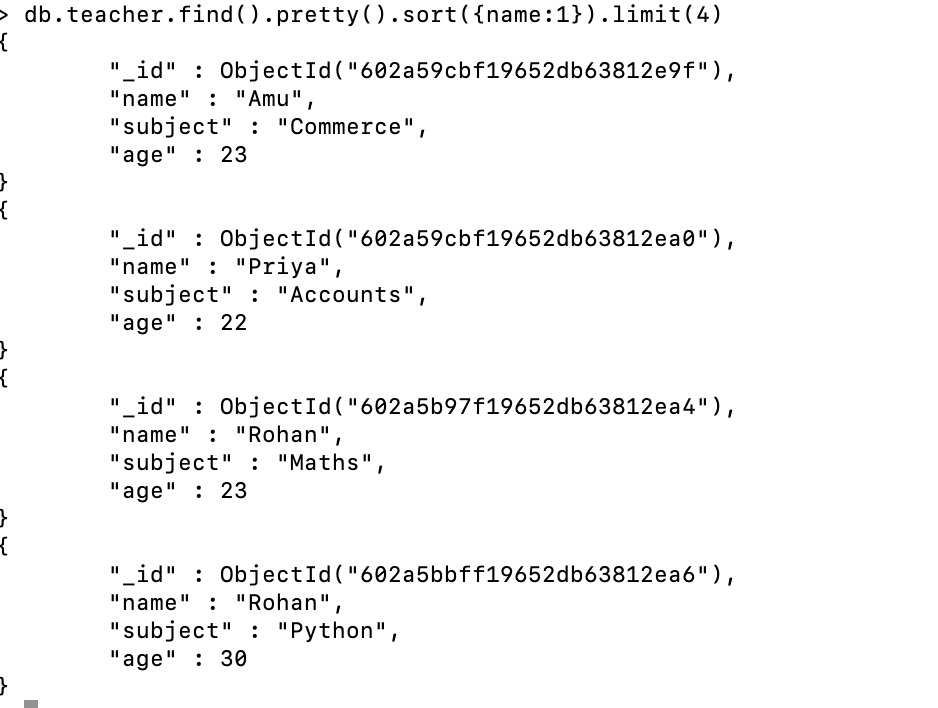In MongoDB, using the sort() method we can sort the metadata values. Let us discuss with the help of an example:

Example:

In the following examples, we are working with:

Database: gfg

Collections: metaexample

Document: Five documents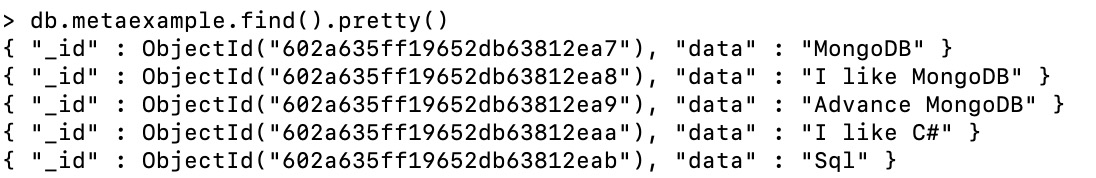Now to sort metadata first we need to create the index using the createIndex() method.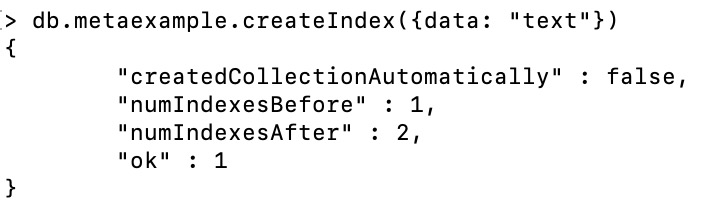```db.metaexample.find({\$text:{\$search:"MongoDB"}},